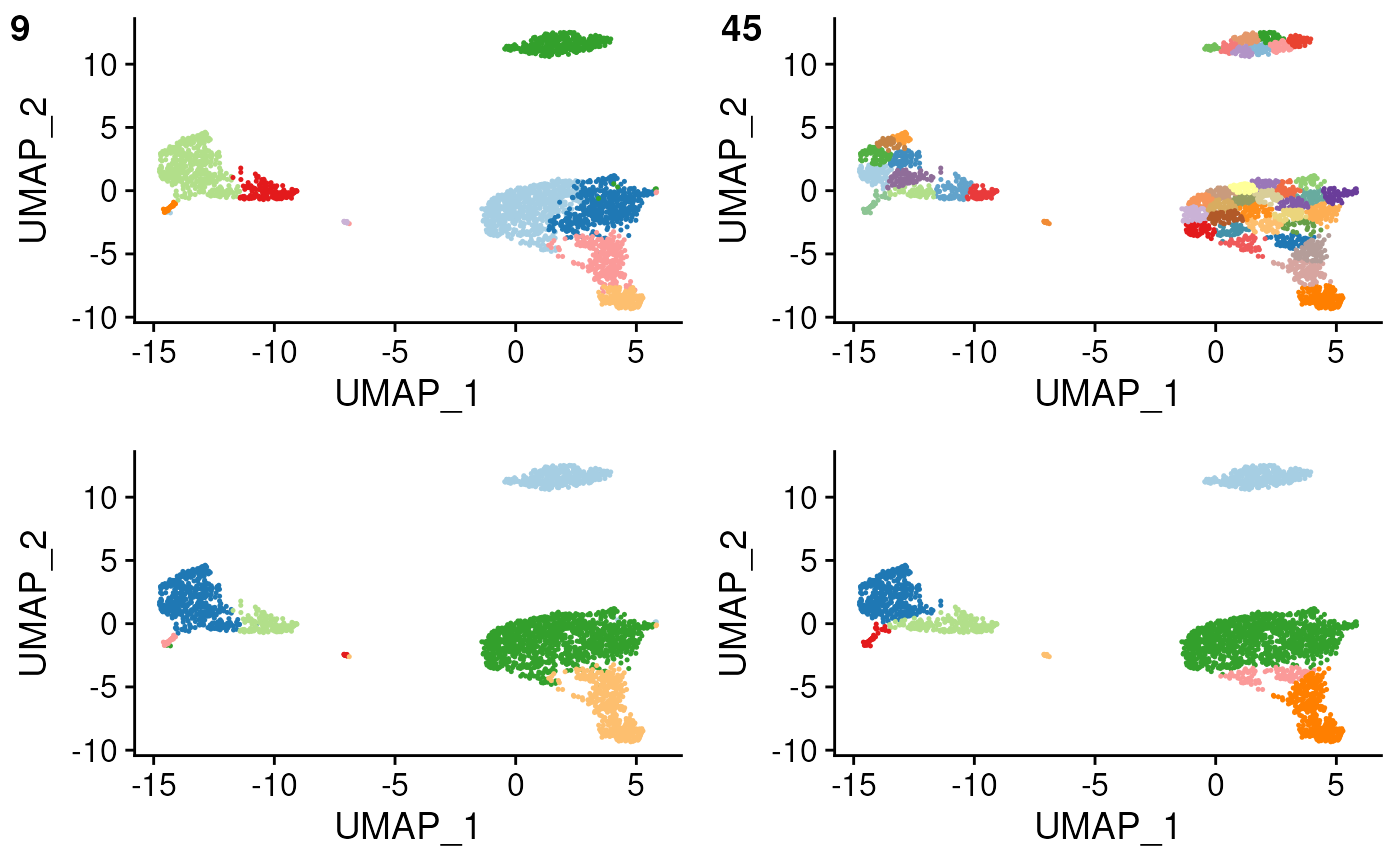compare clustering parameters and classification outcomes

## Usage

overcluster_test(
expr,
ref_mat,
cluster_col,
x_col = "UMAP_1",
y_col = "UMAP_2",
n = 5,
ngenes = NULL,
query_genes = NULL,
threshold = 0,
do_label = TRUE,
do_legend = FALSE,
newclustering = NULL,
combine = TRUE
)

## Arguments

expr

expression matrix

metadata including cluster info and dimension reduction plotting

ref_mat

reference matrix

cluster_col

x_col

column of metadata for x axis plotting

y_col

column of metadata for y axis plotting

n

expand n-fold for over/under clustering

ngenes

number of genes to use for feature selection, use all genes if NULL

query_genes

vector, otherwise genes with be recalculated

threshold

type calling threshold

do_label

whether to label each cluster at median center

do_legend

whether to draw legend

newclustering

use kmeans if NULL on dr or col name for second column of clustering

combine

if TRUE return a single plot with combined panels, if FALSE return list of plots (default: TRUE)

## Value

faceted ggplot object

## Examples

set.seed(42)
overcluster_test(
expr = pbmc_matrix_small,